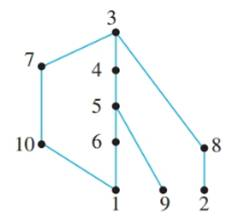Chapter 8.5, Problem 50ES### Discrete Mathematics With Applicat...

5th Edition
EPP + 1 other
ISBN: 9781337694193

#### Solutions

Chapter
Section### Discrete Mathematics With Applicat...

5th Edition
EPP + 1 other
ISBN: 9781337694193
Textbook Problem
1 views

# A set S of jobs can be ordered by writing x ≺ _ y to mean that either x = y or x must he done before y, for all x and y in S. The following is a Hasse diagram for this relation for a particular set S of jobs.a. If one person is to perform all the jobs, one after another, find an order in which the jobs can be done. b. Suppose enough people are available to perform any number of jobs simultaneously. (i) If each job requires one day to perform, what is the least number of days needed to perform all ten jobs? (ii) What is the maximum number of jobs that can be performed at the same time?

To determine

(a)

To find the order in which jobs can be done if one person performs all the jobs one after another.

Explanation

Given information:

A set S of jobs can be ordered by writing xy to mean that either x=y or x must be done before y, for all x and y in S. The following is a Hasse diagram for the relation for the particular set of jobs.

To determine

(b)

To find the least number of days needed to perform all ten jobs if each requires one day to perform and also the maximum number of jobs that can be performed at the same time.

### Still sussing out bartleby?

Check out a sample textbook solution.

See a sample solution

#### The Solution to Your Study Problems

Bartleby provides explanations to thousands of textbook problems written by our experts, many with advanced degrees!

Get Started